Courses

# Units And Dimensional Analysis JEE Notes | EduRev

## JEE : Units And Dimensional Analysis JEE Notes | EduRev

The document Units And Dimensional Analysis JEE Notes | EduRev is a part of the JEE Course Chemistry Class 11.
All you need of JEE at this link: JEE

UNITS AND DIMENSIONAL ANALYSIS: CONVERSION OF UNITS
The simplest way to carry out calculations that involve different units is to use dimensional analysis.
The simplest way to carry out calculations that involve different units is to use dimensional analysis.
As there is a need to convert units from one system to the other. The method used to accomplish this is called factor label method or unit factor method or dimensional analysis.

In this method a quantity expressed in one unit is converted into an equivalent quantity with a different unit by using conversion factor which express the relationship between units: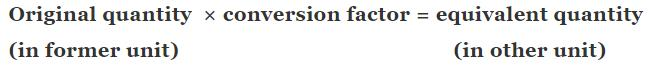This is based on the fact that ratio of each fundamental quantity in one unit with their equivalent quantity in other unit is equal to one For example, in case of mass
1 kg = 2.205 pound = 1000 gm
In this way any derived unit first expressed in dimension and each fundamental quantity like mass length time are converted in other system of desired unit to work out the conversion factor.
For example: How unit of work / energy i.e. joule, in S.I. system is related with unit erg in C.G.S system
Dimension of work = force x displacement = MLT-2 × L = ML2T-2
1 joule = 1 kg (1 metre)2 × (1sec)-2
⇒ 1 × Kg × 1000 gm/1Kg × [1 metre × 100 cm/1 metre] × [1 sec]-2
⇒ 100 gm × (100)2 × 1 em2 × (1 sec)-2
⇒ 1000 × 10000 × 1 gm × 1 cm2 × 1 sec-1
⇒ 1 joule = 107 erg

Similarly, we can deduce other conversion factor for other quantity in different unit by the dimensional analysis method.
Another interesting example is the conversion of liter – atmosphere to joule (the SI unit of energy) by multiplying with two successive unit factors.
Thus,
1 L atm × (10-3 m3/1L) × 101.325 Pa/1 atm
= 101. 325 Pa M3
Knowing that Pa = N/m2,
we can write
101.325 Pa m3 = 101.325 (N/m2) m3
= 101.325 N m = 101.325 J
Hence, 1 L atm = 101.325 J

MASS AND WEIGHT
Mass of a substance is the amount of matter present in it while weight is the force exerted by gravity on an object. The mass of a substance is constant whereas its weight may vary from one place to another due to change in gravity. The mass of a substance can be determined very accurately by using an analytical balance.

Volume
Volume has the units of (length)3. So, volume has units of m3 or cm3 or dm3. A common unit, litre (L) is not an SI unit, is used for measurement of volume of liquids.
1 L = 1000 mL,
1000 cm3 = 1 dm3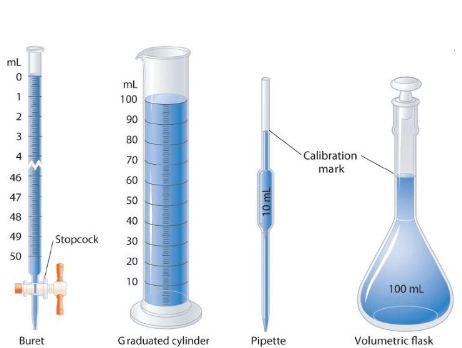Fig: Some volume measuring devices

DENSITY
Density of a substance is its amount of mass per unit volume.SI unit of density = SI unit of mass/SI unit of volume = kg/m3 or kg m–3. This unit is quite large and a chemist often expresses density in g cm–3.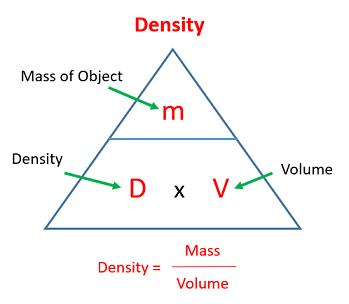Fig: Relationship between density, mass and volumeTEMPERATURE
There are three common scales to measure temperature:-
1) °C (degree Celsius)
2) °F (degree Fahrenheit) and
3) K (kelvin)
Here, K is the SI unit.

Conversion Formulas:
1. K = °C + 273.15

2. °F = (9/5) °C +32

Note — Temperature below 0 °C (i.e. negative values) are possible in the Celsius scale but in the Kelvin scale, the negative temperature is not possible.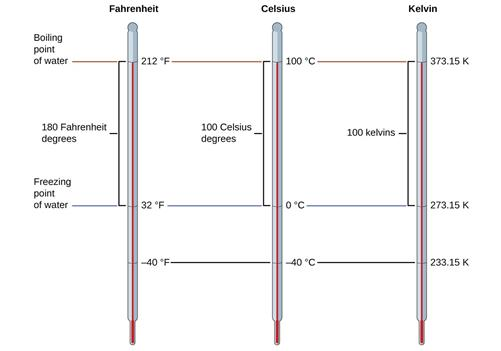Fig: Temperature Kelvin scale

Example 1. What is the mass of 1 L of mercury in grams and in kilograms if the density of liquid mercury is 13.6 g cm−3?
Solution. We know the relationship, 1 L = 1000 cm3 and
Also, density = mass/volume
We can write, mass = (volume) (density)
Therefore, the mass of 1 L of mercury is equal toThe mass in kilograms can be calculated as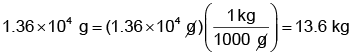(Remember,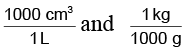are conversion factors with which we have to multiply for getting our answer in appropriate units).

Example 2. How unit of velocity i.e. kilometer/hour is related to unit meter/second.
Solution. We know the relationship, velocity = total displacement/total time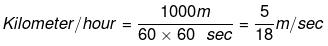Prefixes in SI system

 Name Symbol Quantity yotta Y 1024 zetta Z 1021 exa E 1018 peta P 1015 tera T 1012 giga G 109 mega M 106 kilo k 103 hecto h 102 deca da 10 deci d 10-1 centi c 10-2 miIIi m 10-3 micro M 10-6 nano n 10-9 pico P 10-12 fempto f 10-15 atto a 10-18 zepto z 10-21 yocto y 10-24
Offer running on EduRev: Apply code STAYHOME200 to get INR 200 off on our premium plan EduRev Infinity!

,

,

,

,

,

,

,

,

,

,

,

,

,

,

,

,

,

,

,

,

,

;